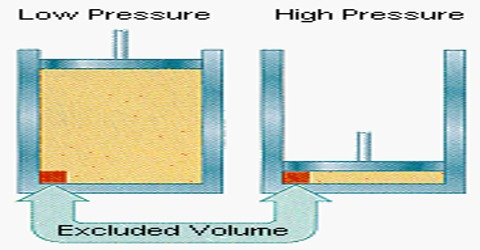# van der Waals’ Equation of State in Terms of Volume Correction

Van der Waals’ Equation of State in Terms of Volume correction

The ideal gas equation has been derived from the kinetic theory of gases with the help of some simplifying assumptions. van der Waals (1873), a Dutch physicist, pointed out that the following two assumptions of the kinetic theory of gases may not be correct. particularly at high pressures and at low temperatures:

(a) the volume of the gas molecules is negligible compared to the volume of the container.

(b) the molecules do not exert any force of attraction on each other.

van der Waals introduced corrections into the ideal gas equation to account for the above assumptions.

Volume correction: The molecules being incompressible themselves, will have a certain volume of their own. This volume may not be negligible when the pressure is very high. A simple calculation will prove this point. Taking the average molecular diameter as 4 x 10-8 cm; the volume of one molecule = 4/3 π (2×10-8)3 mL.  The volume of one mole (Avogadro number of molecules) of the gas is:

4/3 π (2×10-8)3 x 6.023 x 1023 mL

= 19.8 mL

The molar volume of a gas, i c., the volume occupied by one mole of a gas at 1atm pressure and O0 is 22.414 mL. At .0 atmosphere pressure the volume of the molecules themselves, 19.8 mL, a negligible compared to the volume of the container in which the gas is contained. But when the pressure is 1000 atmosphere the latter value becomes 22.414 mL. Compared to this value the volume 19.8 mL of the gas molecules themselves cannot be negligible.

The volume of the gas, by which is meant the free space available to the gas molecules for free movement, is not V, the volume of the vessel, but less than V by a quantity b. At constant temperature, (V – b) and not V, will vary with pressure according to Boyle’s law. It can be seen that b is related to the size of the gas molecules and hence a rough idea of the molecular size may be obtained if b can be evaluated. b is not, however, the actual volume of the molecules, but is about 4 times the actual volume of the molecules.